## Introduction

This document is intended to help users of the `mosaic` package migrate their `lattice` package graphics to `ggformula`. The mosaic package provides a simplified and systematic introduction to the core functionality related to descriptive statistics, visualization, modeling, and simulation-based inference required in first and second courses in statistics.

Originally, the `mosaic` package used `lattice` graphics but now support is also available for the improved `ggformula` system. Going forward, `ggformula` will be the preferred graphics package for Project MOSAIC.

## Histograms

### Histograms (ggformula)

``````library(mosaic)   # also loads ggformula
gf_histogram(~ age, data = HELPrct)``````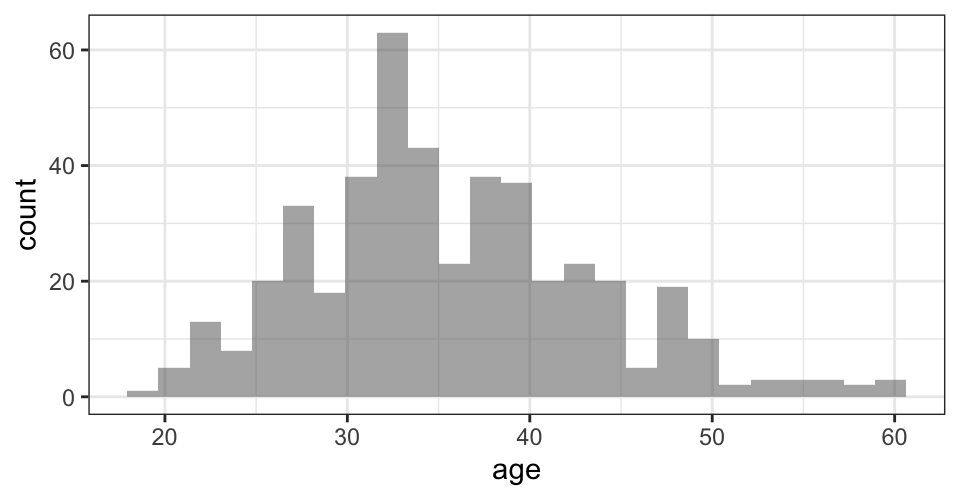### Histogram options (ggformula)

``````gf_histogram(~ age, data = HELPrct,
binwidth = 5) ``````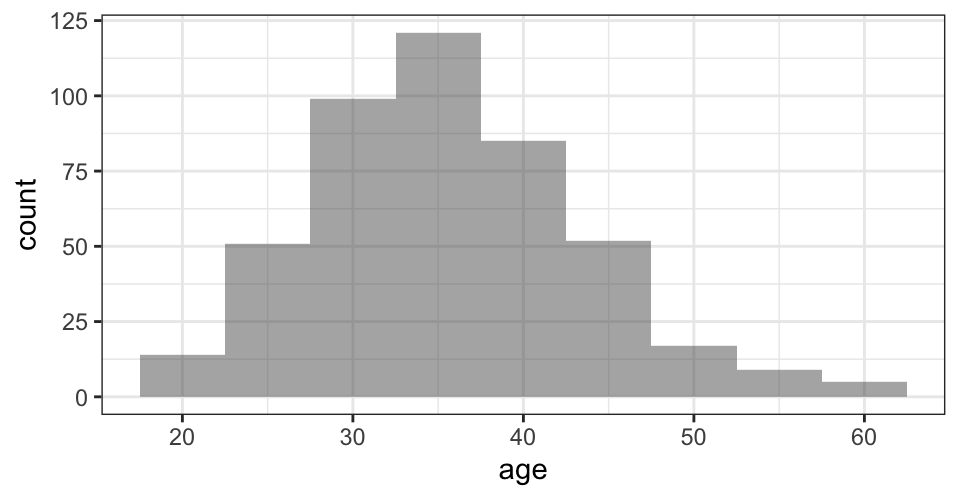### Histograms (lattice)

``````library(mosaic)     # also loads lattice
histogram(~ age, data = HELPrct)``````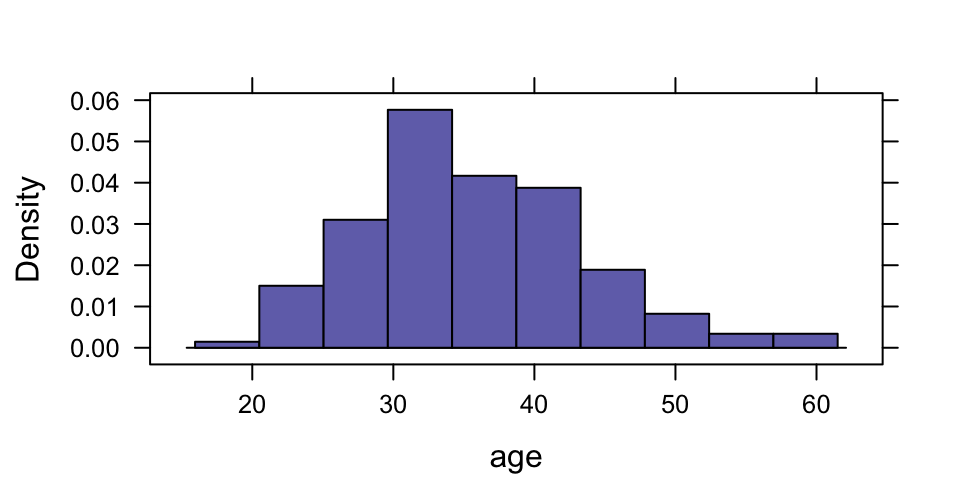### Histogram options (lattice)

``histogram(~ age, width = 5, data = HELPrct)``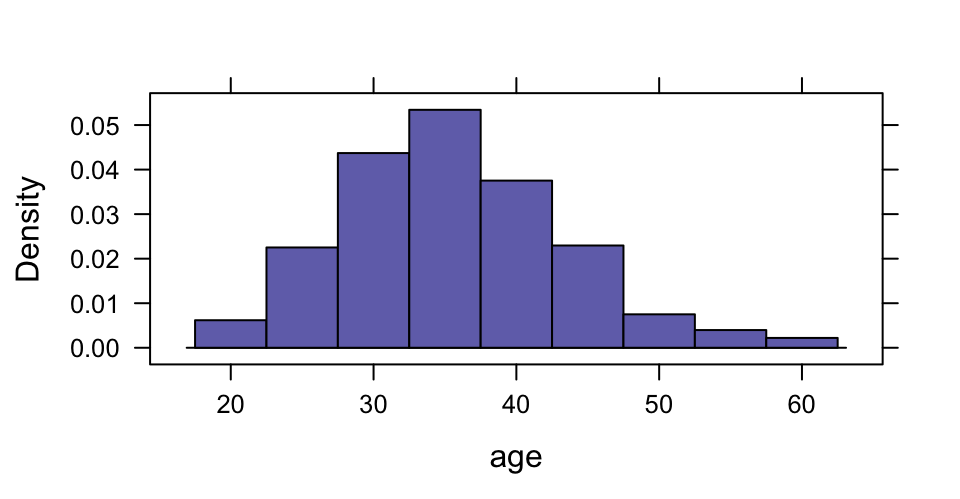## Density Plots

### Density plots (ggformula)

``gf_dens(~ age, data = HELPrct)``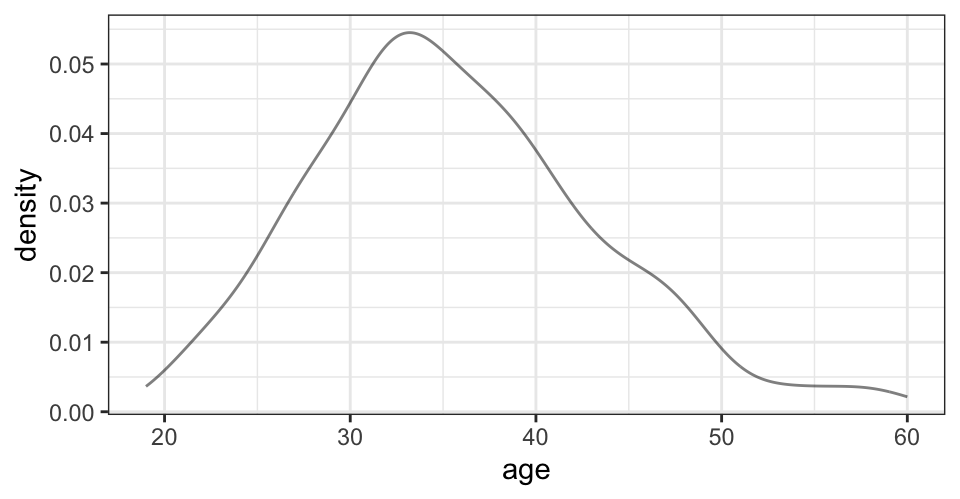### Overlaid density plots (ggformula)

``````gf_dens(~ age, data = HELPrct,
color = ~ sex)``````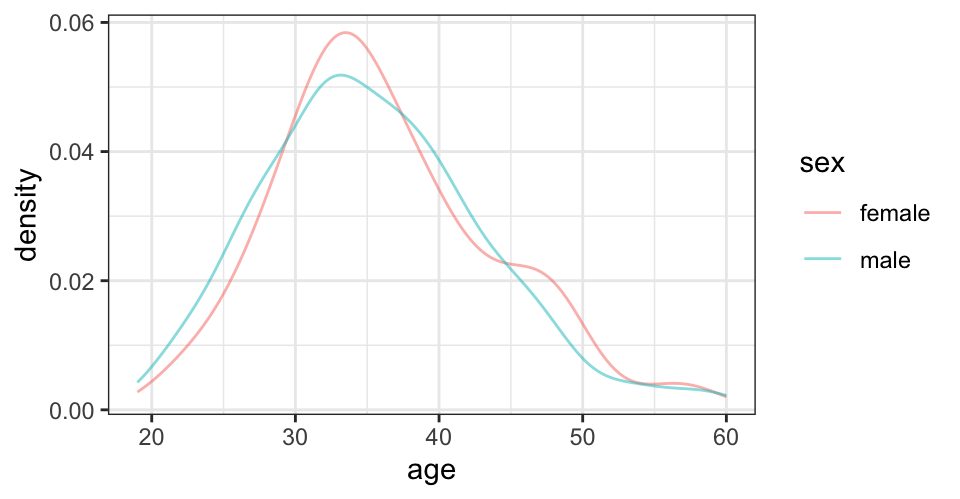### Density over histograms (ggformula)

We can use stacked layers to add a density curve based on a maximum likelihood fit or a kernel density estimate (see also `gf_dist()`)

``````gf_dhistogram( ~ age, data = HELPrct,
alpha = 0.5) %>%
gf_fitdistr(color = ~"MLE", dist = "dnorm") %>%
gf_dens(color = ~"KDE")   ``````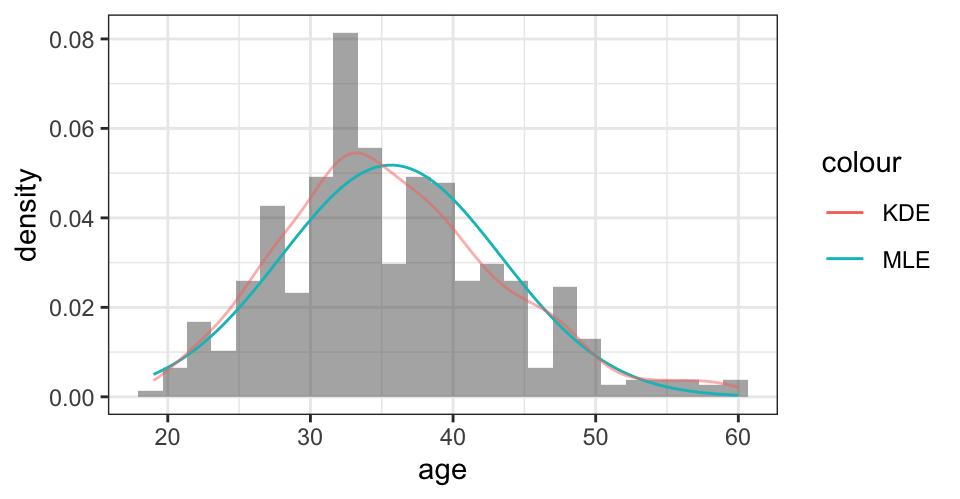### Density plots (lattice)

``densityplot(~ age, data = HELPrct)``